Email us to get an instant 20% discount on highly effective K-12 Math & English kwizNET Programs!

#### Online Quiz (WorksheetABCD)

Questions Per Quiz = 2 4 6 8 10

### Grade 4 - Mathematics8.9 Types of Fractions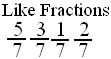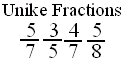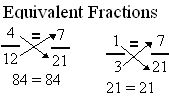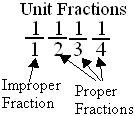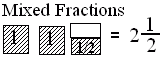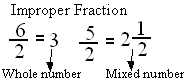Directions: Answer the following questions. Also write at least 5 examples of each type of fractions.
 Q 1: If the numerator is more than the denominator in a fraction, the fraction is called a _________.mixed fractionimproper fractionproper fraction Q 2: Fractions with different denominators are calledunlike fractionslike fractions Q 3: Fractions with same denominators are calledunlike fractionslike fractions Q 4: When a products obtained by cross multiplication give the same number, then the fractions are ____(or equal).equivalentmixedproper Q 5: If the numerator is less than the denominator in a fraction, the fraction is called a _______.proper fractionimproper fractionmixed fraction Q 6: A mixed number denotes the sum of a whole number-part and proper fraction part.FalseTrue Question 7: This question is available to subscribers only! Question 8: This question is available to subscribers only!